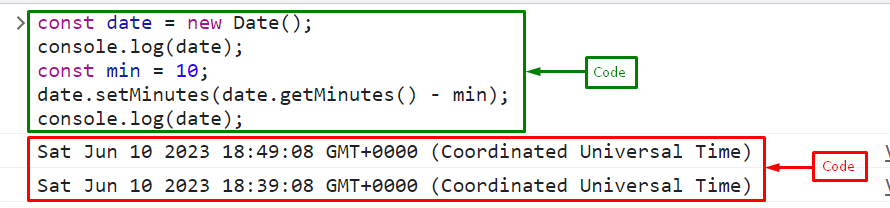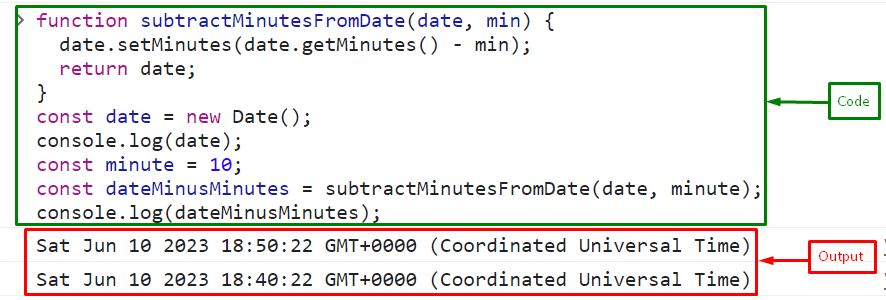# How to Minus/Subtract Minutes From Date in JavaScript?

Working with date and time is common in many JavaScript applications. When working with dates and times in JavaScript, it is often necessary to perform calculations or manipulate the values to meet specific requirements. One common task is subtracting a certain number of minutes from a given date and time. JavaScript provides a rich set of methods for working with Date objects, allowing developers to efficiently perform operations such as subtracting minutes.

This tutorial will describe the method to minus minutes from a date in JavaScript.

## How to Minus/Subtract Minutes From Date in JavaScript?

For subtracting minutes from the date, use the “setMinutes()” with the combination of the “getMinutes()” method of the Date object. By combining the setMinutes() method with the getMinutes() method, developers can easily manipulate dates and perform precise calculations in their applications.

### Example

First, call the date object to get today’s date and then print it on the console:

```const date = new Date();
console.log(date);```

Set the number of minutes to the const type variable “min”:

`const min = 10;`

Subtract the minutes from the Date object by calling the setMinutes() method on the date object. We will call the getMinutes() method inside the setMinutes() method as an argument and subtract the number of minutes from it. This will update the date object to represent the new date and time after subtracting the specified minutes:

```date.setMinutes(date.getMinutes() - min);
console.log(date);```

The output shows that the 10 minutes have been successfully subtracted from the time:You can also define a method for subtracting minutes from the date that will call any time by providing the specified date and minutes to subtract as an argument:

```function subtractMinutesFromDate(date, min) {
date.setMinutes(date.getMinutes() - min);
return date;
}```

To specify the date, pass the date to the Date object. Here, we will call the Date() constructor for getting today’s date and time:

```const date = new Date();

console.log(date);```

Set the number of minutes to the variable “minute”:

`const minute = 10;`

Call the defined function for subtracting minutes from the date by passing the date and minute as arguments and store the result in the variable “dateMinusMinutes”:

`const dateMinusMinutes = subtractMinutesFromDate(date, minute);`

Print the resultant date o the console utilizing the “console.log()” method:

`console.log(dateMinusMinutes);`

OutputThat’s all about the date minus minutes in JavaScript.

## Conclusion

To subtract minutes from the date, use the “setMinutes()” with the combination of the “getMinutes()” method of the Date object. It is a convenient way to subtract minutes from dates. This tutorial described the method for subtracting minutes from a date in JavaScript.